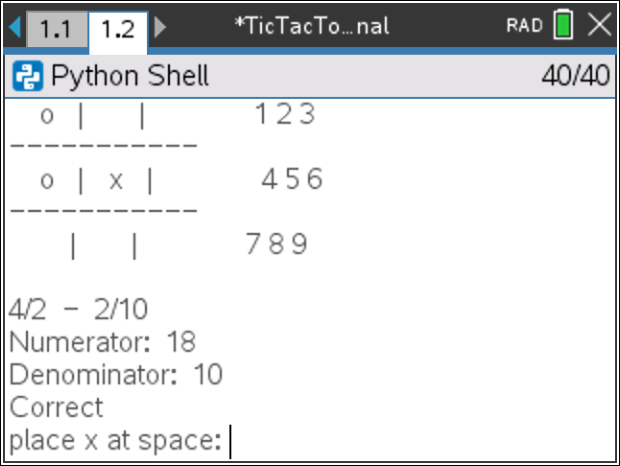# Activities

•• ##### Subject Area

• Math: Explorations with Coding: Coding with Python

6-8

• ##### Device
•TI-Nspire™ CX II
• ##### Software

TI-Nspire™ CX

5.4

## Fraction Tic Tac Toe#### Activity Overview

In this coding activity, students will create a fraction based, tic tac toe game using Python and mathematics.

#### Objectives

• Use variables to store values
• Use the randint() function to generate integers
• Use the print() function to display
• Use a while loop to repeat code.
• Add and subtract fractions with unlike denominators.
• Multiply and divide fractions.(Teacher Note step 9 tells how to restrict to add and subtract)
• Add, subtract, multiple, and divide fractions with positive and negative values. (Teacher note step 3 to restrict to positive values).